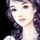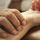## R语言基础

174人评价 查看评价
9.8 内容实用
9.8 简洁易懂
9.5 逻辑清晰
•犀利明
rstudio设置为中文后会不认识jan，只认识中文的一月 > x5 <- "一月 1,2015 02:01" > x6 <- strptime(x5,"%B %d,%Y %H:%M") > x6  "2015-01-01 02:01:00 CST" ＃正确 > x5 <- "Jan 1,2015 02:01" > x7 <- strptime(x5,"%B %d,%Y %H:%M") > x7  NA ＃错误
查看全部
•田园_0001
2.5 factor(因子) --分类数据/有序 vs 无序 --整数向量+标签（label） 创建因子 x<-factor(c("female","female","male","male","female")) y<-factor(c("female","female","male","male","female"),levels = c("male","female")) table(x) unclass(x) class(unclass(x))
查看全部
4 采集 收起

2018-03-22

•江心乱影_XL

R数据结构

2.矩阵（matrix）：向量+维度属性（整数向量：nrow, ncol）

2.1 创建矩阵：

方法一： x <- matrix(nrow=3, ncol=2) #创建一个3行2列矩阵，元素为NA。该函数创建矩阵时先列后行。

x <- matrix(1 : 6, nrow=3, ncol=2) #按列填充元素

方法二： y <- 1 : 6  #生成一个向量

dim(y) <- c(2, 3) #指定行数与列数

其他：dim(matrix)：查看矩阵matrix的行数与列数

attributes(matrix)：查看矩阵matrix有哪些属性

2.2 拼接矩阵

```y1 <- 1 : 6
dim(y1) <- c(2 : 3)
y2 <- matrix(1:6, nrow =2, ncol=3)

rbind(y1, y2) #按行拼接
cbind(y1, y2) #按列拼接```

3.数组：与矩阵类似，但维度可以大于2

创建数组：x <- array(1:24, dim=c(4, 6)) #参数1填充元素，参数2指定维度

y <- array(1:24, dim=c(2, 3, 4))

查看全部
•小Hua
七、总结数据信息 #默认前六行或者后六行 head(airquality, 10)#查看前10行 tail(airquality, 10)#查看后10行 summary(airquality)#总结，数据分布整体把握 str(airquality)#以简洁方式对数据总结 table(airquality\$Month)#对列进行频数统计 table(airquality\$Ozone, useNA = "ifany")#将Ozone中NA的数值统计出来 any(is.na(airquality\$Ozone))#判断是否有缺失值，true是有缺失值 sum(is.na(airquality\$Ozone))#统计缺失值数量 all(airquality\$Month < 12)#查看是不是所有的月份都小于12 #将Titanic强制转换为数据框 t <- as.data.frame(Titanic) x <- xtabs(Freq ~ Class + Age, data = t)#按照Class和Age生成交叉表 ftable(x)#扁平化显示 object.size(airquality)#查看对象大小 print(object.size(airquality),units="Kb")#按照kb显示大小
查看全部
3 采集 收起 来源：总结数据信息

2018-03-22

•小Hua
列表（list）： 1.列表与向量的差别：列表可以同时包含不同类型的对象 2. l <- list("a", 2, 10L, 3+4i, TRUE)#建立列表 l2 <- list(a=1 ,b=2 ,c=3)#为列表元素命名 l3 <- list(c(1,2,3),c(4,5,6,7))#列表元素可以是向量，创建元素个数大于1 x <- matrix(1:6, nrow=2, ncol=3) dimnames(x) <- list(c("a","b"),c("c","d","e"))#为矩阵维度（行、列）命名
查看全部
•i2019
#split #根据因子或者因子列表将向量或其他对象分组 #通常与lapply一起使用 #参数格式：split(向量/列表/数据框,因子/因子列表) x <- c(rnorm(5), runif(5),rnorm(5,1)) f <- gl(3,5) x f split(x,f) lapply(split(x,f), mean) lapply(split(x,f), sum) head(airquality) split(airquality,airquality\$Month)#按照month分组查看 s <- split(airquality,airquality\$Month) s table(airquality\$Month)#查看每个Month下包含的记录数 # 求平均值 lapply(s, function(x) colMeans(x[,c("Ozone","Wind","Temp")])) #简化显示结果 sapply(s, function(x) colMeans(x[,c("Ozone","Wind","Temp")])) #处理缺失值 sapply(s, function(x) colMeans(x[,c("Ozone","Wind","Temp")],na.rm = T))
查看全部
2 采集 收起 来源：R语言函数 split

2018-03-22

•weixin_慕容1489917

向量（vector）：只能包含同一类型的对象

创建向量的方法：1. 定义 x <- vector(数据类型，数据长度)

2. x1 <- 1:4   #创建整型向量1-4

3. x3 <- c(向量的元素)  # 例如构建向量x3<-c(1，2）

#对于向量中元素类型不同的情况，R语言中会自动强制转化

例如x3 <- c(TRUE,10,"a") 会转换为("TRUE","10","a"）

as.numeric(x)   #将x转换为数值型向量

as.logical(x)      #将x转换为逻辑向量

as.character(x)  #将x转换为字符型向量

names(x1) <- c("a","b","c")  #给向量x1进行命名,“a”为第一个向量元

素的名字，依次类推

查看全部
•慕后端1494413

列表：

1、l<-list("a",2,10L,3+4i,TRUE) #列表可以包含各种类型的数据

2、l2<-list(a = 1,b= 2,c = 3)#给列表的元素命名

3、l3<-list(c(1,2,3),c(4,5,6)) #列表中每个元素的内容个数>1

4、x<-matrix(1:6,nrow = 2,ncol = 3) dimnames(x) <- list(c("a","b"),c("c","d","e"))#矩阵的维度只能用列表来引入？？

5、列表和向量都可以包含不同类型的数据，但是列表中的元素可以是向量。

查看全部
•杜仲先生

#排序

```x<-data.frame(v1=1:5,v2=c(10,7,9,6,8),v3=11:15,v4=c(1,1,2,2,1))
x
sort(x\$v2,decreasing = TRUE)#对数据框v2进行降序排列
sort(x\$v3)#对数据框v3进行升序排列
order(x\$v2)#返回的不是内容本身，而是内容对应的下标排序
x[order(x\$v2),]#行顺序按照order方式排列
x[order(x\$v4,x\$v3),]#先排序v4，再排序v3，v4的排序结果更强
x[order(x\$v4,x\$v3,decreasing = TRUE),]#先排序v4，再排序v3，v4的排序结果更强```

查看全部
•杜仲先生

#split函数

```#根据因子或因子列表将向量或其它对分组
#与lapply联用
x <- c(rnorm(5), runif(5),rnorm(5,1))
f<-gl(3,5)
split(x,f)#f因子中的水平1，2，3
lapply(split(x,f),mean）#对列表中得每一个元素求平均值
s<-split(airquality,airquality\$Month)#\$符号代表选择Month
table(airquality\$Month)
lapply(s,function(x)colMeans(x[,c("Ozone","Wind","Temp")]))#s其实是一个数据框；计算平均值时，未将缺失值排除，故得出一些缺失值结果
sapply(s,function(x)colMeans(x[,c("Ozone","Wind","Temp")],na.rm = TRUE))#使用na.rm=TRUE排除缺失值并求平均值，sapply对lapply结果进行了化简```

查看全部
2 采集 收起 来源：R语言函数 split

2018-10-11

•滕玉龙 02:22
> # vectorized > x <-1:5 > y <- 6:10 > x+y  7 9 11 13 15 > x*y  6 14 24 36 50 > x/y  0.1666667 0.2857143 0.3750000 0.4444444 0.5000000 > x <- matrix(1:4,nrow=2,ncol=2) > y <- matrix(req(2,4),nrow=2,ncol=2) Error in matrix(req(2, 4), nrow = 2, ncol = 2) : could not find function "req" > y <- matrix(rep(2,4),nrow=2,ncol=2) > x [,1] [,2] [1,] 1 3 [2,] 2 4 > y [,1] [,2] [1,] 2 2 [2,] 2 2 > x*y [,1] [,2] [1,] 2 6 [2,] 4 8 > x/y [,1] [,2] [1,] 0.5 1.5 [2,] 1.0 2.0 > x %*% y [,1] [,2] [1,] 8 8 [2,] 12 12 >
查看全部
2 采集 收起 来源：向量化操作

2018-03-22

•滕玉龙 00:08
向量化操作：可以作用于向量、矩阵等结构，使得代码简介、易于阅读、效率高。 向量、矩阵之间的加减乘除是依次对应的元素之间做加减乘除。 矩阵之间要进行矩阵乘法：矩阵名1 %*% 矩阵名2 rep(num1,num2) #num1重复num2次
查看全部
2 采集 收起 来源：向量化操作

2017-06-12

•滕玉龙 05:08
x[!is.na(x)] #返回不是缺失值的元素 complete.cases() #()内对应的都不是缺失值的才为TRUE，否则返回的向量中都为FALSE library(datasets) #得到R的数据集 head(数据集名称) #返回数据集前六列的数据 可通过g <- complete.cases(数据集名称)返回所有的结果 数据集名[g,][1:10,] #表示不含有缺失值的，所有变量都显示，显示第1到第10行，显示所有列
查看全部
2 采集 收起 来源：处理缺失值

2018-03-22

•滕玉龙
因子factor：用来处理分类数据的。可以理解为整数向量+标签（label）（优于整数向量，每个数字有自己具体的含义）。常用于线性模型。 分类数据可分为有序与无序。 创建因子： ①因子名字 <- factor(c()) #在c中依次因子包含的内容female,male,female,male... ②因子名字 <- factor(c(),levels=c()) #可通过levels设定基线水平 查看因子：table(因子名字) 去掉标签：unclass(因子名字)
查看全部
2 采集 收起

2018-03-22

•没死接着学
数据框的子集： 数据框名\$列名[编号或c()] #获取数据框中的元素，或者用于矩阵相同的方法获取元素。 通过"数据框名\$列名"来返回对应的向量。 which()返回的是判断语句中为真的对应的下标，而不用which()返回的是TRUE和FALSE的向量 subset(要构建子集的变量名，构建子集的条件) #可对应所有类型的数据
查看全部
2 采集 收起 来源：数据框的子集

2018-03-22

0/150

1、R语言的数据结构 2、构建数据子集 3、重要函数的使用